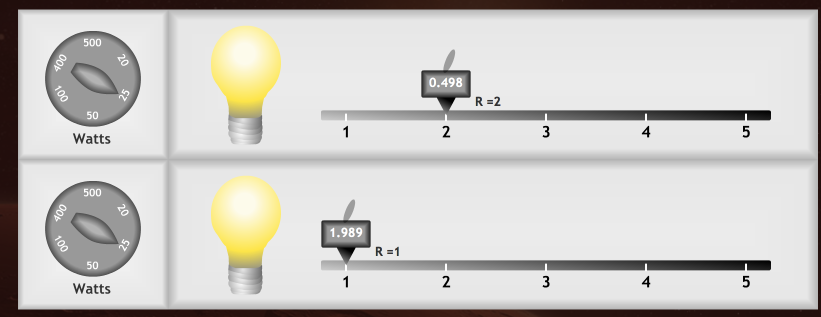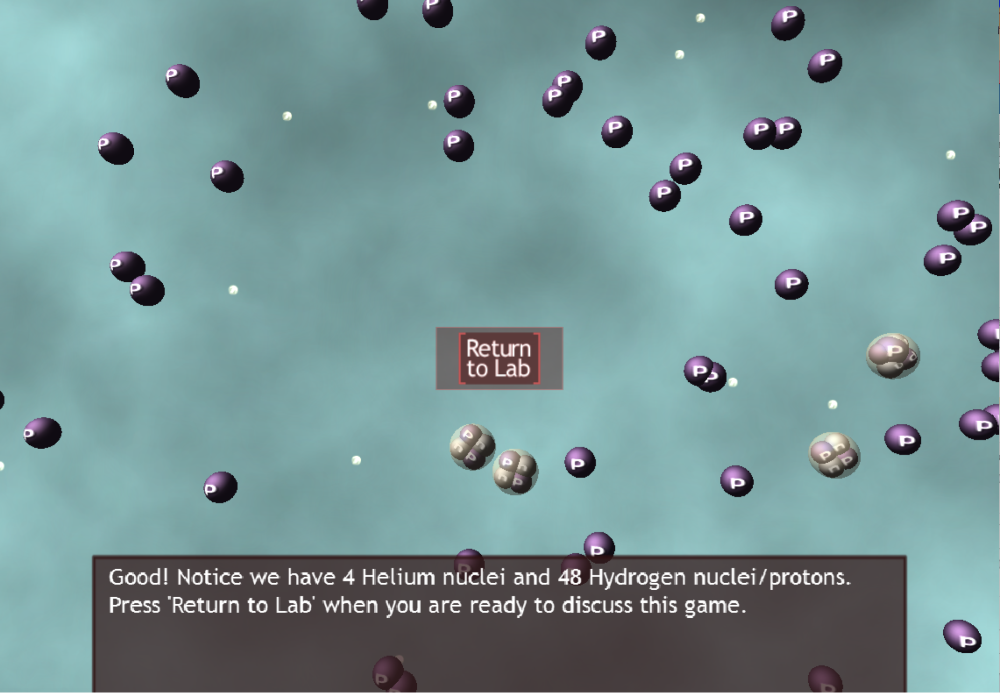# Sample Test QuestionsWhat is the exoplanet orbital period and the percent brightness drop for the case in the image above from the Kepler Exoplanet Transit Hunt activity?

A: 60 days and 0.15%
B: 20 days and 0.15%
C: 10 days and 0.15%
D: 5 days and 0.30%
E: 60 days and 0.30%In the inverse square law activity above, what would the wattage of the top lightbulb need to be such that it appeared as bright as the bottom lightbulb which is a 25 Watt bulb?

A: 400 Watts
B: 100 Watts
C: 50 Watts
D: 25 Watts
E: 20 WattsIn the p-p chain, shown in the above diagram, what particles must be input in order to produce one 4He nucleus?

A: 8 protons, but 2 of them are returned when the 4He is produced
B: 6 protons, but 2 of them are returned when the 4He is produced
C: 2 photons and 2 positrons
D: 2 protons and 4 neutrons
E: 4 neutronsIn what box does the galaxy in the bottom center belong in this Hubble tuning fork diagram?

A: E1
B: Irr
C: Sc
D: SBb
E: SBcIn the figure above, how would you calculate the fraction of the mass of the gas in the universe that is in the form of Helium?

A: take 4x4 for the mass of Helium and divide by 4x4+48x1 for the total mass
B: take 4x1 for the mass of Helium and divide by 48x1 for mass of Hydrogen
C: take 4 for the mass of Helium an divide by 48 for the mass of Hydrogen
D: take 48x1 for the mass of Hydrogen and divide by 4x4+48x1 for total mass
E: take 48x4 for the mass of Hydrogen and divide by 4x1 + 48x4 for total mass